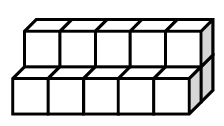### Home > CC2 > Chapter 9 > Lesson 9.2.3 > Problem9-77

9-77.

Find the surface area and volume of the prism shown at right.If each edge measures $1$ unit, then:
1) each side surface has an area of $1$ un2.
2) the volume of one cube is $1$ un3.

How many cubes make up this object?
How many sides make up the surface of the object?

$\text{Surface Area}=46$ square units
$\text{Volume}=15$ cubic units